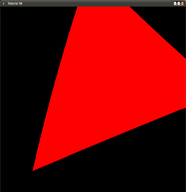modern opengl tutorial

end

# 第七课 旋转变换

## 背景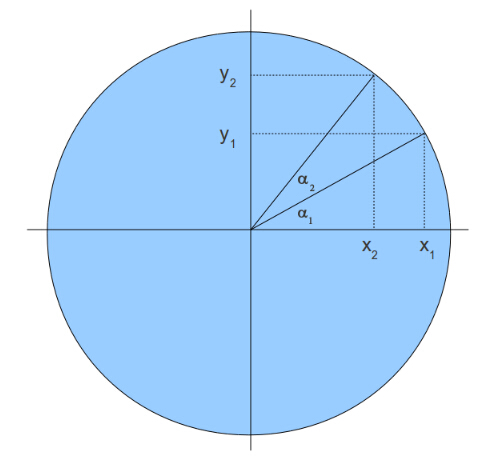x1=cos(a1)
y1=sin(a1)
x2=cos(a1+a2)
y2=sin(a1+a2)

cos(a+b) = cosacosb - sinasinb
sin(a+b) = sinacosb+cosasinb

x2=cos(a1+a2) = cosa1cosa2- sina1sina2 = x1cosa2- y1sina2
y2=sin(a1+a2) = sina1cosa2+ cosa1sina2 = y1cosa2+ x1sina2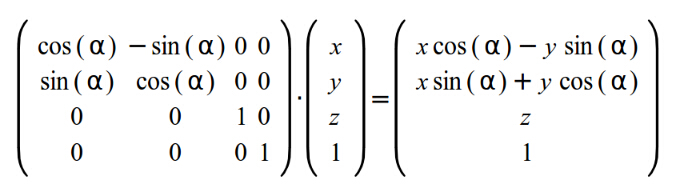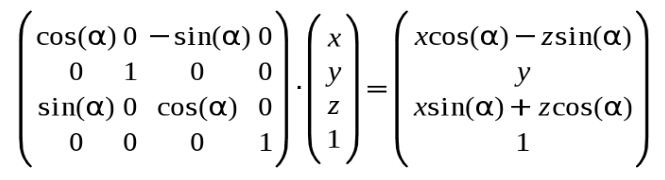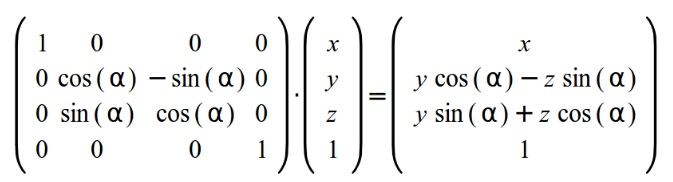## 代码

``````World.m=cosf(Scale);               World.m=-sinf(Scale);World.m=0.0f;　　　　　　　　　　　World.m=0.0f;
World.m=sinf(Scale);　World.m=cosf(Scale);  World.m=0.0f;World.m=0.0f;
World.m=0.0f;       World.m=0.0f;        World.m=1.0f;　　　　　　　World.m=0.0f;
World.m=0.0f;       World.m=0.0f;        World.m=0.0f;　　　　　　　 World.m=1.0f;``````

## 操作结果